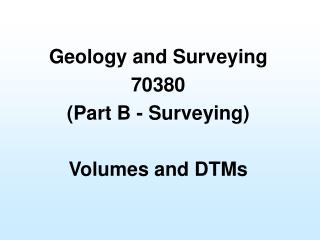# Geology and Surveying 70380 (Part B - Surveying) Volumes and DTMs - PowerPoint PPT PresentationDownload PresentationGeology and Surveying 70380 (Part B - Surveying) Volumes and DTMs

Download Presentation## Geology and Surveying 70380 (Part B - Surveying) Volumes and DTMs

- - - - - - - - - - - - - - - - - - - - - - - - - - - E N D - - - - - - - - - - - - - - - - - - - - - - - - - - -
##### Presentation Transcript

1. Geology and Surveying 70380 (Part B - Surveying) Volumes and DTMs

2. Objectives In this lecture we will look at: • General volume calculations from field information • Trapezoidal Rule for volumes • Simpson’s Rule for volumes • Forming DTMs

3. Volume of a Regular Box V = a × b × c c a b

4. Volume of a Pyramid h b a

5. Volume of a Frustum A1 and A2 are parallel A1 Perpendicular height between A1 and A2 h A2

6. a h If d=a b d Volume of a Wedge h = Vertical Height

7. Volume of a Prismoid A Prismoid is a solid having for its ends any two parallel plane figures, and having plane sides. l A2 A1

8. Trapezoidal Rule for Volumes • Prismoidal Formula • End Area Formula Combine several prismoid formulae. Where n is the last cross-section Convenient since any number of prismoids or cross sections can be used

9. Simpson’s Rule for Volume • Follow similar arguments to Trapezoidal Formula to extend Simpson’s Rule for areas and develop Simpson’s Rule for volumes • Odd number of cross sections at equal distance apart • If even number, calculate last prismoid independently

10. Earthworks Volume • Areas and volumes are included on Cross Sections and Longitudinal Sections • Generally economy suggests a “balance” of cut and fill after topsoil stripping • Mass Haul is also a major consideration to contractors • Study example on page 21.17

11. Grid Leveling h2 h3 h4 h1 a b

12. Grid Leveling h2 h3 h4 h1 b a h5 h6 b a b

13. Grid Leveling h2 h3 h4 h1 h8 b a h5 h6 h7 b a b b

14. Grid Leveling General Formula Appearing once = corners of grid Appearing twice = sides of grid Appearing three times = only irregular shapes Appearing four times = internal points

15. Contours • Contouring is the geographic representation of land forms (shape of land surface) • A contour is a line of constant elevation • Therefore the vertical interval between contours (or contour interval - CI) is constant • Therefore the distance between contours indicates the steepness of grade

16. Example of Contours

17. Levels for Contours and DTM Levels can be taken by several means including: • Grid Leveling (pre-marked grid) • Spot Leveling (Chainage and offset) • Spot Leveling (H angle and distance) • Spot Leveling (GPS) Much better handled by a fully automated surveying sustem – Total Station and data collector or RTK GPS

18. Earthworks Quantities from Contours

19. Digital Terrain Models DTM = Digital Terrain Model DEM = Digital Elevation Model Used by CADD packages

20. Contouring Models • Usually a points modeler (with strings) • Surface represented by E, N and RL of a number of grid or random points • Surface must be defined (usually be triangles – triangular plates) • This assumes a smooth surface between points – Caution! • Triangles are then contoured

21. Points – Spot Levels

22. Triangles

23. Contours from Triangles

24. Contours from Triangles

25. Contours from Triangles

26. Triangles and Contours

27. Contours

28. String Lines • Model represented by points and string lines • Strings used to join common points • Strings also used to define changes of grade (COGs) • These COGs are also known as “Breaklines” • Every string becomes the side of a triangle if that string is a breakline.

29. 101.0 101.0 101.0 102.0 102.0 102.0 101 m Contour 100.0 100.0 100.0 Without Breakline Strings

30. 101 m Contour With Breakline Strings 101.0 101.0 101.0 Breakline 102.0 102.0 102.0 100.0 100.0 100.0

31. Breaklines

32. DTMs for Volume Calculation • Volumes from prisms • Volumes from cross-sections • Volumes from triangles • Make decision on most accurate for each application – then check by alternate method • Volumes can be from a datum surface, or between two surfaces (eg Design surface – Natural Surface)

33. Summary In this lecture we investigated: • General volume calculations from field information • Trapezoidal Rule for volumes • Simpson’s Rule for volumes • Forming DTMs • Significance of Breaklines • CADD applications and volume calcs

34. Self Study • Read Module 21 • Do self assessment Questions

35. Questions?